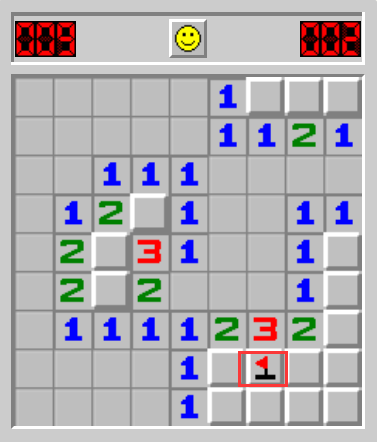# C語言實現經典掃雷小遊戲完整代碼（遞歸展開 + 選擇標記）

## 遊戲介紹## 遊戲整體框架

test.c：遊戲邏輯的測試，包含遊戲菜單的打印，遊戲設計的基本邏輯的展示。

game.c：遊戲功能的具體實現，這部分是整個遊戲的核心代碼，一般不會展示給用戶。

game.h：相關頭文件的包含、符號的聲明以及函數的聲明。

## 遊戲具體功能及實現

### 1、雷盤的定義### 2、雷盤的初始化### 3、佈置雷### 4、排查雷### 5、遞歸式展開一片### 6、獲取周圍雷的個數### 7、標記特定位置### 8、打印雷盤## 遊戲完整代碼

### 1、test.c

```#define _CRT_SECURE_NO_WARNINGS 1
#include"game.h"
{
printf("*****************************************\n");
printf("*********  1.play      0.exit   *********\n");
printf("*****************************************\n");
}
void game()
{
//定義用於存放雷和顯示雷的數組
char mine[ROWS][COLS];
char show[ROWS][COLS];
//數組初始化
BoardInit(mine, ROWS, COLS, '0');
BoardInit(show, ROWS, COLS, '*');
//埋雷
SetMine(mine, ROW, COL);
system("cls");   //清除菜單，美觀整潔
//打印雷盤
//BoardPrint(mine, ROW, COL);   //用於自己調試觀察，在發佈時註釋掉
BoardPrint(show, ROW, COL);
//排雷
FindMine(mine, show, ROW, COL);
}
int main()
{
//設置隨機數的種子
srand((unsigned int)time(NULL));
int input = 0;
do
{
printf("請選擇：");
scanf("%d", &input);
switch (input)
{
case 1:
game();
break;
case 0:
printf("退出遊戲!\n");
break;
default:
printf("輸入錯誤，請重新輸入!\n");
break;
}
} while (input);
return 0;
}
```

### 2、game.h

```#pragma once
#include<stdio.h>
#include<windows.h>
#include<time.h>
#include<stdlib.h>
#define ROW 9
#define COL 9
#define ROWS ROW + 2
#define COLS COL + 2
#define MINE_COUNT 10
//數組初始化
void BoardInit(char board[ROWS][COLS], int rows, int cols, char set);
//埋雷
void SetMine(char board[ROWS][COLS], int row, int col);
//排雷
void FindMine(char mine[ROWS][COLS], char show[ROWS][COLS], int row, int col);
//打印雷盤
void BoardPrint(char board[ROWS][COLS], int row, int col);
```

### 3、game.c

```#define _CRT_SECURE_NO_WARNINGS 1
#include"game.h"
//數組初始化
void BoardInit(char board[ROWS][COLS], int rows, int cols, char set)
{
int i = 0;
int j = 0;
for (i = 0; i < rows; i++)
{
for (j = 0; j < cols; j++)
{
board[i][j] = set;   //set表示要初識化的字符
}
}
}
//埋雷
void SetMine(char board[ROWS][COLS], int row, int col)
{
int count = MINE_COUNT;
while (count)
{
int x = rand() % row + 1;      //隨機生成雷的坐標
int y = rand() % col + 1;
if (board[x][y] == '0')        //檢查該位置是否已經有雷
{
board[x][y] = '1';
count--;
}
}
}
//打印雷盤
void BoardPrint(char board[ROWS][COLS], int row, int col)
{
int i = 0;
int j = 0;
printf("------掃雷遊戲------\n");
for (i = 0; i <= row; i++)   //打印行號
printf("%d ", i);
printf("\n");
for (i = 1; i <= row; i++)
{
printf("%d ", i);   //打印列號
for (j = 1; j <= col; j++)
{
printf("%c ", board[i][j]);
}
printf("\n");
}
printf("------掃雷遊戲------\n");
}
//標記雷的位置
void MarkMine(char board[ROWS][COLS], int row, int col)
{
int x = 0;
int y = 0;
while (1)
{
printf("請輸入你想要標記位置的坐標->");
scanf("%d %d", &x, &y);
if (x >= 1 && x <= row && y >= 1 && y <= col)    //判斷該坐標是否合法
{
if (board[x][y] == '*')        //判斷該坐標是否被排查
{
board[x][y] = '!';
break;
}
else
{
printf("該位置不能被標記，請重新輸入!\n");
}
}
else
{
printf("輸入錯誤，請重新輸入!\n");
}
}
}
//獲取坐標周圍雷的個數
int GetMineCount(char board[ROWS][COLS], int x, int y)
{
int i = 0;
int j = 0;
int count = 0;
for (i = x - 1; i <= x + 1; i++)
{
for (j = y - 1; j <= y + 1; j++)
{
if (board[i][j] == '1')
{
count++;
}
}
}
return count;
}
//遞歸爆炸式展開一片
void ExplosionSpread(char mine[ROWS][COLS], char show[ROWS][COLS], int row, int col, int x, int y, int* pw)
{
if (x >= 1 && x <= row && y >= 1 && y <= col)  //判斷坐標是否為排查范圍內
{
int num = GetMineCount(mine, x, y);   //獲取坐標周圍雷的個數
if (num == 0)
{
(*pw)++;
show[x][y] = ' ';   //如果該坐標周圍沒有雷，就把該坐標置成空格，並向周圍八個坐標展開
int i = 0;
int j = 0;
for (i = x - 1; i <= x + 1; i++)
{
for (j = y - 1; j <= y + 1; j++)
{
if (show[i][j] == '*')    //限制遞歸條件，防止已經排查過的坐標再次遞歸，從而造成死遞歸
ExplosionSpread(mine, show, row, col, i, j, pw);
}
}
}
else
{
(*pw)++;
show[x][y] = num + '0';
}
}
}
//排雷
void FindMine(char mine[ROWS][COLS], char show[ROWS][COLS], int row, int col)
{
int x = 0;
int y = 0;
int win = 0;  //用來標記是否取得勝利
int* pw = &win;
char ch = 0;   //用來接受是否需要標記雷
while (win < row * col - MINE_COUNT)
{
printf("請輸入你想要排查的坐標->");
scanf("%d %d", &x, &y);
if (x >= 1 && x <= row && y >= 1 && y <= col)   //判斷坐標合法性
{
if (mine[x][y] == '1')
{
system("cls");
printf("很遺憾，你被炸死瞭!\n");
BoardPrint(mine, row, col);   //被炸死瞭就打印mine數組，讓用戶知道自己怎麼死的
break;
}
else
{
if (show[x][y] != '*')   //判斷是否重復排查
{
printf("該坐標已被排查，請重新輸入!\n");
continue;  //直接進入下一次循環
}
else
{
ExplosionSpread(mine, show, row, col, x, y, pw);  //爆炸展開一片
system("cls");  //清空屏幕
BoardPrint(show, row, col);  //打印棋盤
printf("需要標記雷的位置請輸入y/Y,否則請按任意鍵->");
while ((ch = getchar()) != '\n');  //清理緩沖區
scanf("%c", &ch);
if (ch == 'Y' || ch == 'y')
{
MarkMine(show, row, col);   //標記雷
system("cls");
BoardPrint(show, row, col);
}
else
{
continue;
}
}
}
}
else
{
printf("輸入錯誤，請重新輸入!\n");
}
}
if (win == row * col - MINE_COUNT)
{
system("cls");
printf("恭喜你，排雷成功!\n");
BoardPrint(show, row, col);
return;
}
}
```

## 遊戲效果展示